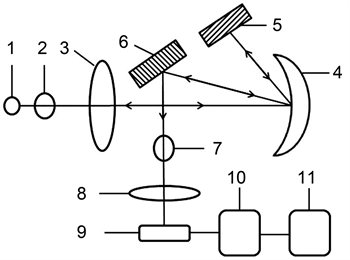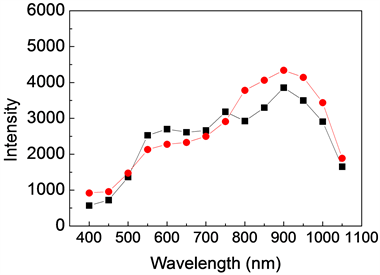﻿ 硅光电二极管的光谱响应测量及其响应时间研究

# 硅光电二极管的光谱响应测量及其响应时间研究Study on Measurement of Spectral Response and Response Time of Silicon Photodiodes

Abstract: The spectral response of Silicon photodiodes with incident light wavelengths of 400 nm to 1050 nm is measured when Silicon photodiodes do not reach maximum response in the background light with no external light, steady air flow and temperature, and halogen lighting. The influence of the relevant conditions on the spectral response of Silicon photodiodes and the laws of the spectral response of Silicon photodiodes are analyzed. The response time of Silicon photodiodes is measured by different resistance and bias voltages during the light measuring, and the physical characteristics of Silicon photodiodes are explained.

1. 引言

${R}_{v}\left(\lambda \right)=\frac{V\left(\lambda \right)}{P\left(\lambda \right)}$ (1)

${R}_{i}\left(\lambda \right)=\frac{I\left(\lambda \right)}{P\left(\lambda \right)}$ (2)

2. 光谱响应装置Figure 1. Schematic of measuring device for spectral response of silicon photodiode

3. 光谱响应度分析

$P\left(\lambda \right)={V}_{f}\left(\lambda \right)R\left(\lambda \right)$ (3)

$P\left(\lambda \right)=\frac{{V}_{f}\left(\lambda \right)}{{R}_{f}{K}_{f}}$ (4)

$R\left(\lambda \right)=\frac{V\left(\lambda \right)}{P\left(\lambda \right)}=\frac{{V}_{b}\left(\lambda \right)/{K}_{b}}{{V}_{f}\left(\lambda \right)/{R}_{f}{K}_{f}}$ (5)Figure 2. Measurement curve of twice spectral responses

4. 硅光电二极管的时间响应测试分析

$A\left(\omega \right)=\frac{1}{{\left(1+{\omega }^{2}{\tau }^{2}\right)}^{1/2}}$ (6)

$V\left(\omega \right)=\frac{{V}_{0}}{{\left(1+{\omega }^{2}{\tau }^{2}\right)}^{1/2}}$ (7)

${V}_{0}$ 表示的是当入射光的调制频率为零时的输出电压。

$\tau =\frac{1}{2\pi }\sqrt{\frac{{V}_{1}^{2}-{V}_{2}^{2}}{{\left({V}_{2}{f}_{2}\right)}^{2}-{\left({V}_{1}{f}_{1}\right)}^{2}}}$ (8)Table 1. Response time of silicon photodiode with different resistance and bias voltages

5. 总结

2019年海南省基础与应用基础研究计划(自然科学领域)高层次人才项目(2019RC190，2019RC192)，海南省自然科学基金(2018CXTD336，618QN241)，海南省高等学校科学研究项目(Hnky2020ZD-12，Hnky2020-24)，海南省重大科技计划项目(ZDKJ2019005)，国家自然科学基金项目(61864002，61964007)和中国工程科技发展战略项目(19-HN-XZ-07)资助。

NOTES

*通讯作者。

 朱世国, 周积骏. 半导体光电二极管伏安特性的实验测定[J]. 物理实验, 2000, 20(2): 12-14.

 崔亦飞, 王洪涛, 张雷. 硅光电二极管光谱响应率的测量[J]. 大学物理实验, 2005, 18(3): 61-63.

 王巍, 陈婷, 李俊峰, 何雍春, 王冠宇, 唐政维, 袁军, 王广. 高光电探测效率CMOS单光子雪崩二极管器件[J]. 光子学报, 2017, 46(8): 0823001-1-0823001-7.

 张燕, 曾光宇, 洪志刚. 硅PIN光电二极管探测系统的研究[J]. 核电子学与探测技术, 2008, 28(2): 391-393.

 赵庆添, 杨连生, 耿秀珍. 半导体光电探测器件光谱响应和响应度的测试[J]. 半导体光电, 1980(4): 35-39.

 张学骜, 吴昊, 张海良, 王飞, 贾红辉, 硅光电二极管光谱响应度的分析[J]. 大学物理实验, 2011, 24(2): 25-27.

 富容国, 常本康, 钱芸生, 詹启海. PIN光电二极管探测器响应特性测试[J]. 光学与光电技术, 2007, 5(1): 11-13.

 初宁, 曹立军, 黄威. 基于光照强度的PIN光电二极管响应时间分析[J]. 传感技术学报, 2013, 26(1): 34-37.

Top